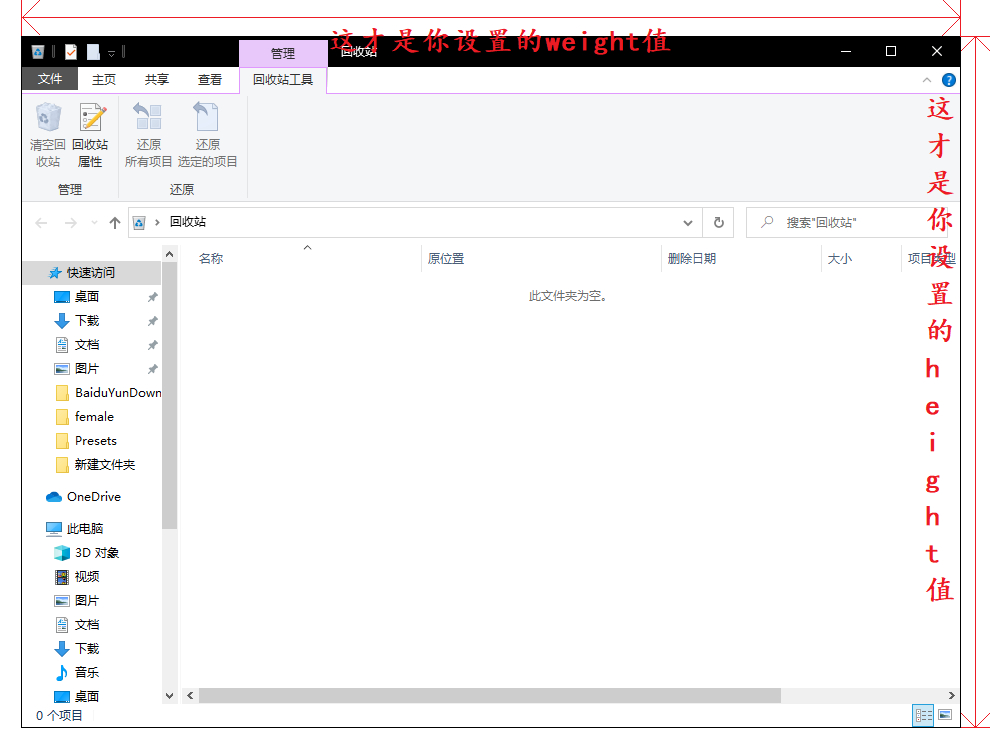``````JFrame()
``````
##### 创建一个无标题的窗口
``````JFrame(String title)
``````
##### 创建标题为title的窗口
``````public voidsetBounds(int x,int y,int width,int height)

``````
##### 设置窗口的初始位置是(x,y)

``````public void setSize(int width,int height)
``````
##### 设置窗口的大小
``````public void setLocation(int x,int y)
``````
##### 设置窗口的位置

``````public void setVisible(boolean b)
``````
##### 设置窗口是否可见

``````public voidsetResizable(boolean b)
``````
##### 设置窗口是否可调整大小

``````publicvoid setExtendedState(int state)
``````
##### 设置窗口的扩展状态

``````    MAXIMIZED_HORIZ //(水平方向最大化)，

MAXIMIZED_VERT //(垂直方向最大化)，

MAXIMIZED_BOTH //(水平、垂直方向都最大化)。
``````
``````publicvoid setDefaultCloseOperation(int operation)
``````
##### 设置关闭图标功能

``````    DO_NOTHING_ON_CLOSE//(什么也不做)，

HIDE_ON_CLOSE //(隐藏当前窗口)，

DISPOSE_ON_CLOSE //(隐藏当前窗口，并释放窗体占有的其他资源)，
EXIT_ON_CLOSE //(结束窗口所在的应用程序)。
``````

# 注意

JFrame的窗口大小是整个窗口的大小，包括外面那一圈，具体看图：Q.E.D.